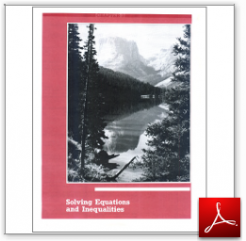Algebra Math Book - Introductory Algebra Thank you for your continual support.

 The power of mathematics rests in the logic of thinking.ID: Chapter03
Price: 9.95

# Algebra Math book - Introductory Algebra - Chapter 3 - Solving Equations and Inequalities

## Chapter 3 of this algebra math book has 8 sections.

To get started, you might like to take a free test just to see what areas you might need to study.  Click on this link.

 Sec3.1 Section3.1-Solving Using the Addition Principle and Multiplication Principle Sec3.2 Section3.2-Combining the Principles to Solve Equations Sec3.3 Section3.3-Solving Equations Containing Parentheses Sec3.4 Section3.4-Solving Word Problems Sec3.5 Section3.5-Applied Problems Sec3.6 Section3.6-Formulas Sec3.7 Section3.7-Solving Inequalities Using the Addition Principle Sec3.8 Section3.8-Solving Inequalities Using the Multiplication Principle

For a complete listing of the objectives in this chapter visit the List of Objectives.

This section of my algebra math book, Introductory Algebra, also includes in the download:

• Cover Sheet